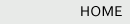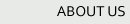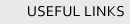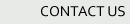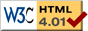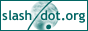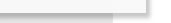## HOW TO CALCULATE.

### PRACTICAL RULES, SHORT METHODS, AND PROBLEMS USED IN BUSINESS COMPUTATIONS.

Rapidity and accuracy in making estimates and in figuring out the result of business transactions is of the greatest necessity to the man of business. A miscalculation may involve the loss of hundreds or thousands of dollars, in many cases, while a slow and tedious calculation involves loss of time and the advantage which should have been seized at the moment. It is proposed in the following pages to give a few brief methods and practical rules for performing calculations which occur in every-day transactions among men, presuming that a fair knowledge of the ordinary rules of arithmetic has previously been attained.

### ADDITION.

To be able to add up long columns of figures rapidly and correctly is of great value to the merchant. This requires not only a knowledge of addition, but in order to have a correct result, one that can be relied upon, it requires concentration of the mind. Never allow other thoughts to be flitting through the mind, or any outside matter to disturb or draw it away from the figures, until the result is obtained. Write the tens to be carried each time in a smaller figure underneath the units, so that afterwards any column can be added over again without repeating the entire operation. By the practice of addition the eye and mind soon become accustomed to act rapidly, and this is the art of addition. Grouping figures together is a valuable aid in rapid addition, as we group letters into words in reading.

 862 \ 538 / 674 \ 843 / ____ 2917

Thus, in the above example, we do not say 3 and 4 are 7 and 8 are 15 and 2 are 17, but speak the sum of the couplet, thus 7 and 10 are 17, and in the second column, 12 and 9 are 21. This method of grouping the figures soon becomes easy and reduces the labor of addition about one-half, while those somewhat expert may group three or more figures, still more reducing the time and labor, and sometimes two or more columns may be added at once, by ready reckoners.

Another method is to group into tens when it can be conveniently done, and still another method in adding up long columns is to add from the bottom to the top, and whenever the numbers make even 10, 20, 30, 40 or 50, write with pencil a small figure opposite, 1, 2, 3, 4 or 5, and then proceed to add as units. The sum of these figures thus set out will be the number of tens to be carried to the next column.

 62 2 8 3 52 41 2 8 4 9 6 2 72 1 82 8 32 5 5 2 7 11 3 21 5 8 8 _________________ 5 0 2 8

### SHORT METHODS OF MULTIPLICATION.

For certain classes of examples in multiplication short methods may be employed and the labor of calculation reduced, but of course for the great bulk of multiplications no practical abbreviation remains. A person having much multiplying to do should learn the table up to twenty, which can be done without much labor.

To multiply any number by 10, 100, or 1000, simply annex one, two, or three ciphers, as the case may be. If it is desired to multiply by 20, 300, 5000, or a number greater than one with any number of ciphers annexed, multiply first by the number and then annex as many ciphers as the multiplier contains.

TABLE.

5 cents equal 1/20 of a dollar.
10 cents equal 1/10 of a dollar.
12-1/2 cents equal 1/8 of a dollar.
16-2/3 cents equal 1/6 of a dollar.
20 cents equal 1/5 of a dollar.
25 cents equal 1/4 of a dollar.
33-1/3 cents equal 1/3 of a dollar.
50 cents equal 1/2 of a dollar.

Articles of merchandise are often bought and sold by the pound, yard, or gallon, and whenever the price is an equal part of a dollar, as seen in the above table, the whole cost may be easily found by adding two ciphers to the number of pounds or yards and dividing by the equivalent in the table.

Example. What cost 18 dozen eggs at 16-2/3c per dozen?

 6 ) 1 8 0 0 _ _ _ _ _ \$ 3 . 0 0

Example. What cost 10 pounds butter at 25c per pound?

 4 ) 1 0 0 0 _ _ _ _ _ \$ 2 . 5 0

Or, if the pounds are equal parts of one hundred and the price is not, then the same result may be obtained by dividing the price by the equivalent of the quantity as seen in the table; thus, in the above case, if the price were 10c and the number of pounds 25, it would be worked just the same.

Example. Find the cost of 50 yards of gingham at 14c a yard.

 2 ) 1 4 0 0 _ _ _ _ _ \$ 7 . 0 0

When the price is one dollar and twenty-five cents, fifty cents, or any number found in the table, the result may be quickly found by finding the price for the extra cents, as in the above examples, and then adding this to the number of pounds or yards and calling the result dollars.

Example. Find the cost of 20 bushels potatoes at \$1.12-1/2 per bushel.

 8 ) 2 0 0 0 2 5 0 _ _ _ _ _ \$ 2 2 . 5 0

If the price is \$2 or \$3 instead of \$1, then the number of bushels must first be multiplied by 2 or 3, as the case may be.

Example. Find the cost of 6 hats at \$4.33-1/3 apiece.

 3 ) 6 0 0 4 _ _ _ _ _ _ 2 4 . 0 0 2 . 0 0 _ _ _ _ _ _ \$ 2 6

When 125 or 250 are multipliers add three ciphers and divide by 8 and 4 respectively.

To multiply a number consisting of two figures by 11, write the sum of the two figures between them.

Example. Multiply 53 by 11. Ans. 583.

If the sum of the two numbers exceeds 10 then the units only must be placed between and the tens figure carried and added to the next figure to the left.

Example. Multiply 87 by 11. Ans. 957.

### FRACTIONS.

Fractional parts of a cent should never be despised. They often make fortunes, and the counting of all the fractions may constitute the difference between the rich and the poor man. The business man readily understands the value of the fractional part of a bushel, yard, pound, or cent, and calculates them very sharply, for in them lies perhaps his entire profit.

#### TO REDUCE A FRACTION TO ITS SIMPLEST FORM.

Divide both the numerator and denominator by any number that will leave no remainder and repeat the operation until no number will divide them both.

Example. The simplest form of 36/45 is found by dividing by 9 = 4/5.

To reduce a whole number and a fraction, as 4-1/2, to fractional form, multiply the whole number by the denominator, add the numerator and write the result over the denominator. Thus, 4 X 2 = 8 + = 9 placed over 2 is 9/2.

#### TO ADD FRACTIONS.

Reduce the fractions to like denominators, add their numerators and write the denominator under the result.

Example. Add 2/3 to 3/4.

2/3 = 8/12, 3/4 = 9/12, 8/12 + 9/12 = 17/12 = 1-5/12. Ans.

#### TO SUBTRACT FRACTIONS.

Reduce the fractions to like denominators, subtract the numerators and write the denominators under the result.

Example. Find the difference between 4/5 and 3/4.

4/5 = 16/20, 3/4 = 15/20, 16/20 - 15/20 = 1/20. Ans.

#### TO MULTIPLY FRACTIONS.

Multiply the numerators together for a new numerator and the denominators together for a new denominator.

Example. Multiply 7/8 by 5/6.

7/8 x 5/6 = 35/48. Ans.

#### TO DIVIDE FRACTIONS.

Multiply the dividend by the divisor inverted.

Example. Divide 7/8 by 5/6.

7/8 X 6/5 = 42/40. Reduced to simple form by dividing by 2 is 21/20 = 1-1/20. Ans.

#### TO MULTIPLY MIXED NUMBERS.

When two numbers are to be multiplied, one of which contains a fraction, first multiply the whole numbers together, then multiply the fraction by the other whole number, add the two results together for the correct answer.

Example. What cost 5-1/3 yards at 18c a yard?

 1 8 c 5 - 1 / 3 _ _ _ _ _ _ 1 8 x 5 = 9 0 1 8 x 1 / 3 = 6 _ _ _ _ _ _ 9 6 c

When both numbers contain a fraction,

First, multiply the whole numbers together,

Second, multiply the, lower whole number by the upper fraction;

Third, multiply the upper whole number by the lower fraction;

Fourth, multiply the fractions together;

Fifth, add all the results for the correct answer.

Example. What cost 12-2/3 pounds of butter at 18-3/4c per pound?

 1 8 - 3 / 4 1 2 - 2 / 3 _ _ _ _ _ _ _ _ 1 8 x 1 2 = 2 1 6 1 2 x 3 / 4 = 9 1 8 x 2 / 3 = 1 2 3 / 4 x 2 / 3 = 6 / 1 2 = 1 / 2 _ _ _ _ _ _ _ _ \$ 2 . 3 7 - 1 / 2

Common fractions may often be changed to decimals very readily, and the calculations thereby made much easier.

#### TO CHANGE COMMON FRACTIONS TO DECIMALS.

Annex one or more ciphers to the numerator and divide by the denominator.

Example. Change 3/4 to a decimal. Ans..75.

We add two ciphers to the 3, making it 300, and divide by 4, which gives us.75. In the same way 1/2 =.5, or 3/4 =.75, and so on. When a quantity is in dollars and fractions of a dollar, the fractions should always be thus reduced to cents and mills.

Home | A to Z | About | Contact Us | Related Links